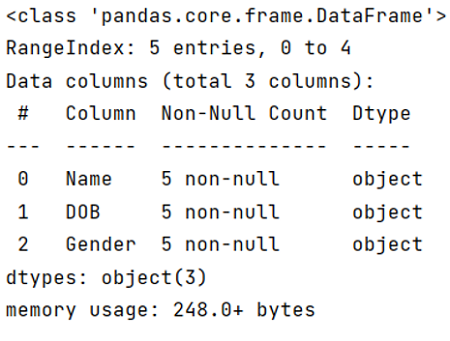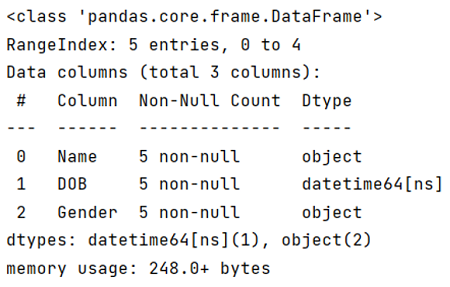# Convert DataFrame column type from string to datetime

Learn how to convert DataFrame column type from string to datetime?
Submitted by Pranit Sharma, on April 26, 2022

Pandas is a special tool that allows us to perform complex manipulations of data effectively and efficiently. Inside pandas, we mostly deal with a dataset in the form of DataFrame. DataFrames are 2-dimensional data structures in pandas. DataFrames consists of rows, columns, and the data. The Data inside the DataFrame can be of any type. Here, we will learn how to convert data in string format into DateTime format.

To convert a string into DateTime, we will use pd.to_datetime() method.

## pandas.to_datetime() Method

This method is used to convert the string into the datetime format. When a CSV file is loaded or when a DataFrame is created then the date is created in string format, this method converts this string data into the correct format.

Syntax:

```pandas.to_datetime(
arg,
errors='raise',
dayfirst=False,
yearfirst=False,
utc=None,
format=None,
exact=True,
unit=None,
infer_datetime_format=False,
origin='unix',
cache=True
)

# short form
pandas.to_datetime(args)
```

Parameter(s):

• It takes the string which has to be converted into the DateTime format.
• It also takes some optional arguments like dayfirst, yearfirst, utc, format.

To work with Python Pandas, we need to import the pandas library. Below is the syntax,

```import pandas as pd
```

Let us understand with the help of an example.

```# Importing pandas package
import pandas as pd

# Creating a Dictionary
dict = {'Name':['Amit','Bhairav','Chirag','Divyansh','Esha'],
'DOB':['07/12/2001','08/11/2002','09/10/2003','10/09/2004','11/08/2005'],
'Gender':['Male','Male','Male','Male','Female']}

# Creating a DataFrame
df = pd.DataFrame(dict)

# Checking datatype of column DOB
print(df.info())

# Converting the column DOB value into datatime format
df['DOB']= pd.to_datetime(df['DOB'])

# Checkig format of each column
print(df.info())
```

Output:At first the datatype of each column is object:After converting the format of DOB column, the datatype has been converted to datetime format.

Preparation